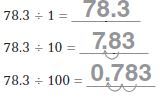Homework Explained - Math Practice 101Dear guest, you are not a registered member. As a guest, you only have read-only access to our books, tests and other practice materials.

As a registered member you can:

Registration is free and doesn't require any type of payment information. Click here to Register.
Go to page:
Chapter 5; Lesson 1:Division Patterns with Decimals

## Division Patterns with Decimals

Complete the pattern.

• Question 1

##•  179 ÷ 100 = 179 ÷ 101 = 179 ÷ 102 = 179 ÷ 103 =
•  87.5 ÷ 100 = 87.5 ÷ 101 = 87.5 ÷ 102 =
•  124 ÷ 1 = 124 ÷ 10 = 124 ÷ 100 = 124 ÷ 1,000 =
•  18 ÷ 1 = 18 ÷ 10 = 18 ÷ 100 = 18 ÷ 1,000 =
•  23 ÷ 100 = 23 ÷ 101 = 23 ÷ 102 = 23 ÷ 103 =
•  51.8 ÷ 1 = 51.8 ÷ 10 = 51.8 ÷ 100 =
•  49.3 ÷ 100 = 49.3 ÷ 101 = 49.3 ÷ 102 =
•  32.4 ÷ 100 = 32.4 ÷ 101 = 32.4 ÷ 102 =
• Question 10

The local café uses 510 cups of mixed vegetables to make 1,000 quarts of beef barley soup. Each quart of soup contains the same amount of vegetables. How many cups of vegetables are in each quart of soup?

• Question 11

The same café uses 18.5 cups of flour to make 100 servings of pancakes. How many cups of flour are in one serving of pancakes?

Yes, email page to my online tutor. (if you didn't add a tutor yet, you can add one here)